# High School Math : Understanding Integrals of Sums, Quotients, and Products

## Example Questions

### Example Question #1 : Introduction To Integrals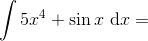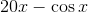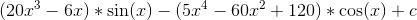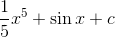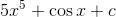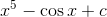Explanation:

Since we're adding terms, we take the integral or anti-derivative of each part separately. For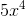, we can use the reverse power rule, which states that we raise the exponent of the variable by one and then divide by that new exponent. For sine, we use our trigonometric integral rules.

Remember,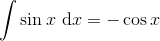.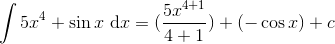Don't forget to include a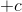to account for any constants!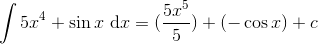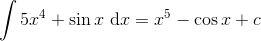### All High School Math Resources